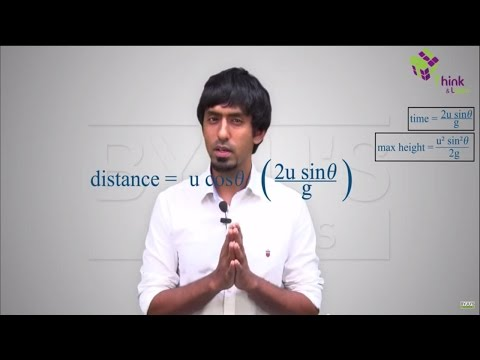Defend yourself better by mastering the science of immunity and vaccines. Secure your free spot, now! Defend yourself better by mastering the science of immunity and vaccines. Secure your free spot, now!

# Uniformly Accelerated Motion - Constant Acceleration

## Uniformly Accelerated MotionHow do you think the motion in which acceleration is constant will proceed? Constant acceleration means velocity changing at a constant rate since the rate of change of velocity is acceleration (first equation of motion).

## Uniform Acceleration Equations

When we are talking about motion in a straight line with constant acceleration, there are three equations of motion, which are helpful in determining one of the unknown parameters:

$$\begin{array}{l}v = u + at\end{array}$$
$$\begin{array}{l}x = ut + \frac{1}{2}at^{2} \end{array}$$
$$\begin{array}{l}v^2 = u^2 + 2as\end{array}$$

where,

v = final velocity of the particle

u = initial velocity of the particle

s = displacement of the particle

a = acceleration of the particle

t = time interval in which the particle is in consideration
Simple algebraic method, graphical method and calculus method are the ways to derive equations of motion.

Remember, the sign convention is to be maintained while applying these equations. One direction is considered to be positive and another one as negative. One of the common examples of uniformly accelerated motion is freely falling bodies. The only acceleration which is acting on the body is g (acceleration due to gravity). If we are taking the vertically upward direction as the positive, the acceleration due to gravity (g) will be negative since it is in the downward direction.

### Uniformly Accelerated Motion In A Plane

Projectile motion is one of the most popular examples of motion in a plane with uniform acceleration. In the case of projectile motion, the only acceleration acting on the particle is the acceleration due to gravity (g). In horizontal direction, the velocity in x-direction will be constant since there is no acceleration. Therefore, we can apply the equations of motion separately in two directions to get results.

In general, a uniformly accelerated motion is the one in which the acceleration of the particle throughout the motion is uniform. It can be moved in one dimension, two dimensions, or three dimensions.

A general case for a body executing uniformly accelerated motion is explained with the help of projectile motion in the video below.

$$\begin{array}{l}~~~~~~~~~~~~~~~\end{array}$$## Frequently Asked Questions – FAQs

Q1

### What is uniform motion?

This type of motion is defined as the motion of an object in which the object travels in a straight line and its velocity remains constant along that line as it covers equal distances in equal intervals of time, irrespective of the duration of the time.
Q2

### What is non-uniform motion?

This type of motion is defined as the motion of an object in which the object travels with varied speed and it does not cover the same distance in equal time intervals, irrespective of the time interval duration.
Q3

### What is centripetal acceleration ?

When an object is moving in a circle and its acceleration vector is pointed towards the centre of that circle, it is known as centripetal acceleration. The unit of centripetal acceleration is m/s2.
Q4

### What is average velocity?

Average velocity is a vector quantity. Average velocity is defined as the change in position or displacement (∆x) divided by the time intervals (∆t) in which the displacement occurs.The average velocity can be positive or negative depending upon the sign of the displacement. The SI unit of average velocity is metres per second (m/s or ms-1).
Q5

### What is uniform acceleration?

When an object is travelling in a straight line with an increase in velocity at equal intervals of time, then the object is said to be in uniform acceleration. Free falling of an object is an example of uniform acceleration.